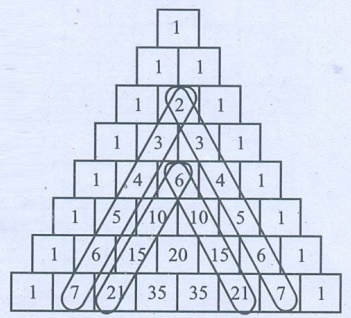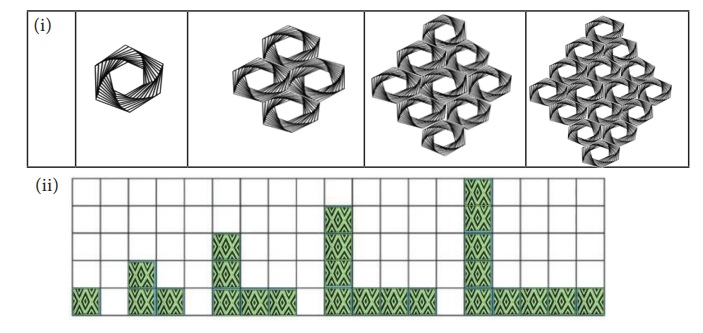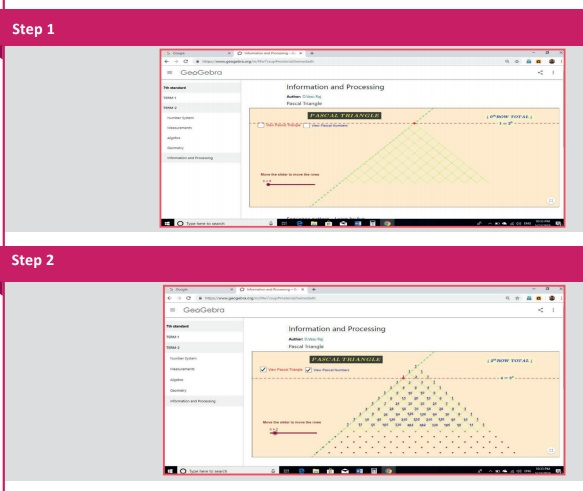Home | | Maths 7th Std | Exercise 5.3

# Exercise 5.3

7th Maths : Term 2 Unit 5 : Information Processing : Miscellaneous Practice problems, Challenge Problems, Text Book Back Exercises Questions with Answers, Solution

Exercise 5.3

Miscellaneous Practice problems

1. Choose the correct relationship between x and y from the given table.(i) y = x+4

(ii) y = x + 5

(iii) y = x + 6

(iv) y = x + 7

Answer: (iii) y = x + 6

2. Find the trianglular numbers from the Pascalâ€™s Triangle and colour them.Solution:3. Write the first five numbers in the third slanting row of the Pascalâ€™s Triangle and find their squares. What do you infer?

Solution:

The first five numbers in the third slanting rows are 1, 3, 6,10,15 .

Their squares 1, 9, 36,100, 225.

Challenge Problems

4. Tabulate and find the relationship between the variables (x and y) for the following patterns.Solution:The relationship between x and y is,

y = x2The relationship between x and y is,

y = 2x â€“ l

5. Verify whether the following hexagonal shapes form a part of the Pascalâ€™s Triangle.Solution:

i) 1 Ă— 13 Ă— 66 = 858

11 Ă— 1 Ă— 78 = 858

1 Ă— 13 Ă— 66 = 11 Ă— 1 Ă— 78

ii) 5 Ă— 21 Ă— 20 = 2100

10 Ă— 8 Ă— 35 = 2100

5 Ă— 21 Ă— 20 = 10 Ă— 6 Ă— 35

iii) 8 Ă— 45 Ă— 84 = 30240

28 Ă— 9 Ă— 120 = 30240

8 Ă— 45 Ă— 84 = 28 Ă— 9 Ă— 120

iv) 56 Ă— 210 Ă— 126 = 1481760

70 Ă— 84 Ă— 252 = 1481760

56 Ă— 210 Ă— 126 = 70 Ă— 84 Ă— 252

These hexagonal shapes form a part of the Pascal's Triangle.

Exercise 5.3

1. (iii) y = x + 6

2.3. Five numbers in third slanting rows are 1, 3, 6, 10, 15 ; Their squares are 1, 9, 36, 100, 225.

Challenge Problems

4. (i) steps (x) 1 2 3 4

shapes (y) 1 4 9 16

(ii) steps (x) 1 2 3 4 5

shapes (y) 1 3 5 7 9

5. (i) 1 Ă— 13 Ă— 66 = 11 Ă— 1 Ă— 78

(ii) 5 Ă— 21 Ă— 20 = 10 Ă— 6 Ă— 35

(iii) 8 Ă— 45 Ă— 84 = 28 Ă— 9 Ă— 120

(iv) 56 Ă— 210 Ă— 126 = 70 Ă— 84 Ă— 252

ICT Corner

Expected Result is shown in this pictureStep-1 :

Open the Browser type the URL Link given below (or) Scan the QR Code. GeoGebra work sheet named â€śInformation and Processingâ€ť will open. There are two activities named â€śPascal Triangleâ€ť and â€ś Sequence pattern-Learn by funâ€ť

Step-2 :

1. In pascal triangle move the slider to scroll over each row and check the row total. 2. In sequence pattern move each slider a, n and i to generate the pattern.